Groundwater Outflow
This method category is dependent upon the selection of the Single Computed Outflow in the Solution Type category. The user-selectable methods in this category are used to calculate Storage and Outflow from the Groundwater object based on a given Inflow.None
This is the default user method for the Groundwater Outflow category. It performs no calculations. If it is selected, RiverWare gives an error because it is unable to calculate either an Outflow from or a Storage for the Groundwater Storage object.Table Flow
The Table Flow method calculates the outflow based on the previous timestep’s Storage and a table input by the user.
Outflow is calculated by a linear interpolation of the Storage Outflow Table, using the Storage calculated at the previous timestep.
Slots Specific to This Method
Storage Outflow Table
Type: TableSlot
Units: VOLUME vs. FLOW
Description: outflow for any given volume of storage
Information:
I/O: Required inputLinear Flow
The Linear Flow method calculates Outflow as a linear function of the previous timestep’s Storage value.
Outflow is calculated by the empirical equation: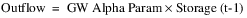Slots Specific to This Method
GW Alpha Param
Type: TableSlot
Units: PER TIME
Description: an empirical parameter used in the Outflow equation
Information:
I/O: Required inputCurrent Storage Linear Flow
The Current Storage Linear Flow method calculates Outflow as a linear function of the Average Storage at the current timestep.
Slots Specific to This Method
Average Storage
Type: SeriesSlot
Units: VOLUME
Description: the average storage over the timestep
I/O: Output only
GW Alpha Param
Type: TableSlot
Units: PER TIME
Description: an empirical parameter used in the Outflow equation
I/O: Required input
Method Details
At each timestep, the method first solves for instantaneous storage, then Average Storage, and then Outflow. The equations are as follows.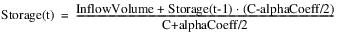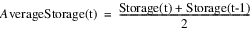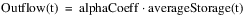Lagged Linear Flow
The Lagged Linear Flow method calculates Outflow linearly as a function of the alpha coefficient times some previous storage value.
Slots Specific to This Method
GW Alpha Param
Type: TableSlot
Units: PER TIME
Description: an empirical parameter used in the Outflow equation
I/O: Required input
Lag Time
Type: TableSlot
Units: TIME
Description: the amount of time lag between a Storage and its corresponding Outflow
I/O: Required input
Method Details
Outflow is computed as follows: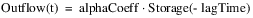Depending on the length of the lag time, one or more storage values may be required prior to the required initial storage, to compute the first outflows. If these values are not input by the user, the method assumes that storage has been constant and uses the initial storage value, which is always required user input.
This method uses instantaneous end-of-timestep storage values.Exponential Flow
The Exponential Flow method calculates Outflow as an exponential function of the previous timestep’s Storage value.
Note:  This calculation is carried out in user units. The value set in the GW Beta Param slot, as set by the user, must be consistent with the user units for the Storage slot. Changing the user units on the Storage slot will produce a different result. There will not be an automatic conversion of the GW Beta Param value.
Slots Specific to This Method
GW Alpha Param
Type: TableSlot
Units: PER TIME
Description: an empirical parameter used in the Outflow equation
Information:
I/O: Required input
GW Beta Param
Type: TableSlot
Units: NONE
Description: an empirical parameter used in the Outflow equation
Information: This value must be consistent with the user units for the Storage slot. If the user units on the Storage slot are changed, the value in this slot must be changed manually to correspond to the new units. Otherwise different results will be produced.
I/O: Required input
Method Details
Outflow is calculated by the empirical equation: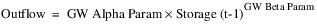The exponential term is computed in user units. That is, Storage(t‑1) is converted to user units, raised to the power of GW Beta Param, and then converted back to the standard RiverWare units before being multiplied by GW Alpha Param).Impulse Response
The Impulse Response method calculates Outflow using a set of impulse response outflow coefficients.
Slots Specific to This Method
Impulse
Type: SeriesSlot
Units: FLOW
Description: the impulse is the inflow minus pumped flow
I/O: Output only
Number of Outflow Coeffs
Type: TableSlot
Units: NONE
Description: the number of impulse response coefficients to use
Information: This single integer value is the number of values that need to be entered in the Outflow Coeffs slot.
I/O: Required input
Outflow Coeffs
Type: TableSlot
Units: NONE
Description: the impulse response coefficients
Information: The same number of coefficients must be input as the value in the Number of Outflow Coeffs slot. The input will be in rows.
I/O: Required input
Method Details
This method first calculates the impulse to groundwater as a function of inflow and pumped flow: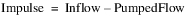Outflow is computed as a function of current and previous impulse values and the outflow coefficients: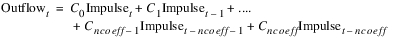Revised: 01/11/2023